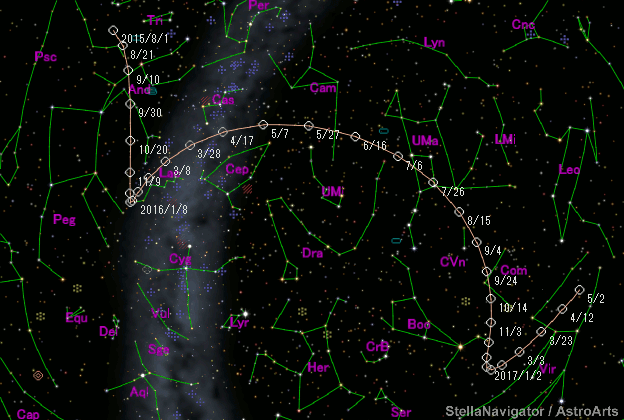# \$B;g6b;3WB@1(B

C/2017 E2 ( Tsuchinshan )###\$B%W%m%U%#!<%k(B

 \$BH/8+F|(B 2017\$BG/(B3\$B7n(B1\$BF|(B \$BH/8+8wEY(B 19.7\$BEy(B \$BH/8+ H. B. Zhao, B. Li, G. Zhaori, R. Q. Hong, L. F. Hu (XuYi\$B!JL\\$X\$s\$KP2!"L\\$X\$s\$KBf!K(B Station, \$B;g6b;3E7J8Bf(B)

###\$B###\$B50F;MWAG(B

```Epoch 2016 May 12.0 TT = JDT 2457520.5
T 2016 May 11.98591 TT                                  MPCW
q   2.3523700            (2000.0)            P               Q
n   0.01001924     Peri.   89.40314     -0.02744174     -0.98004669
a  21.3098100      Node    11.56221     -0.21992472     -0.18619365
e   0.8896109      Incl.   79.17315     +0.97513080     -0.06957300
P  98.37
From 134 observations 2017 Mar. 1-May 29, mean residual 0".4.
```

###\$B@1?^(B###\$B8wEYJQ2=(B

```        m1 = 11.0 + 5 log\$B&\$(B + 10.0 log r
```##### \$B50F;MWAG\$O(BM.P.E.C. 2017-L52\$B\$K7G:\\$5\$l\$?\$b\$N\$G\$9!#(B \$B@1?^\$O%9%F%i%J%S%2!<%?(B Ver.10 (\$B%"%9%H%m%"!<%D(B) \$B\$G:n@.\$7\$?\$b\$N\$G\$9!#(B \$B8wEY%0%i%U\$O(BComet for Windows\$B\$G:n@.\$7\$?\$b\$N\$G\$9!#(B